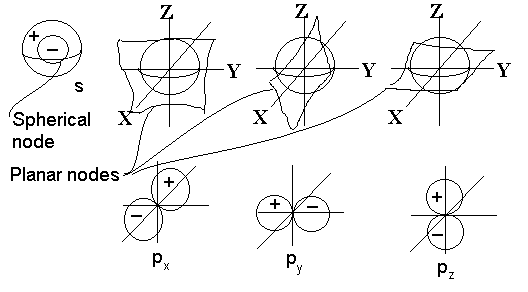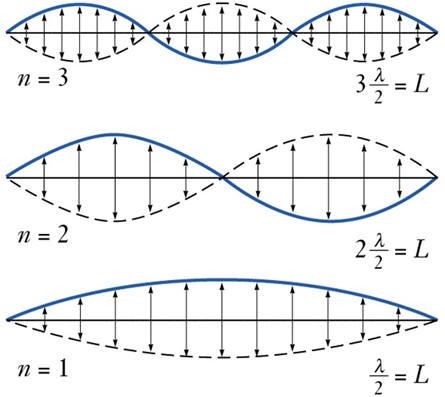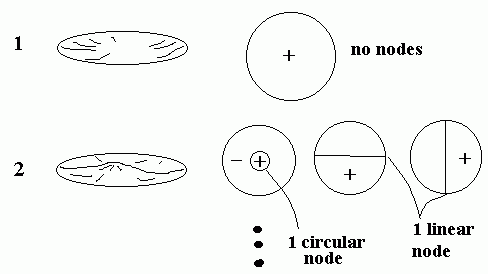# Spherical Harmonics and Quantum numbersAtomic orbitals are calculated from mathematical wave functions that predict the probability of finding an electron or a pair of electrons in a specific region around the atom’s nucleus. Quantum mechanics tells us that electrons in an atom possess four quantum numbers that determine what energy level the electron is at. They are: Orbital, n=1,2,3…; Azimuthal, l=0,1,2…n−1; Magnetic, ml=−l,−l+1…0…l−1,l; and finally Spin, ms=−1/2,1/2. The first three quantum numbers determine location, the last, spin, is a property of the electron itself.

To understand the location quantum numbers, we move onto harmonics. First in one dimension modelled as a guitar string, then two dimensions as the surface of a drum, then in three dimensions as the surface of a balloon or spherical shell.

###In One Dimension

If the string vibrates with one continuous motion over it’s whole length, then it is vibrating in the lowest energy mode.  We have a standing wave with length of 2l.  If the string is prevented from vibrating in certain positions (nodes) the string will be forced to vibrate in a different mode. Each of these modes differs by the number of nodes in the wave function and in the frequency that results. (Listen).  Note that to excite a particular mode of vibration, it is best to pluck the strung near the maximum in the vibrational wave function.

### In Two DimensionsDid you ever listen to a steel drum player?  By tapping on different parts of the drum surface, (s)he can make different notes sound out of the instrument.  That’s because there are carefully placed dents in the surface of the drum that tends to damp out certain vibrations and which set up vibrational modes in the surface with maxima located in different places around the surface. By tapping in the location of a maximum of a particular vibrational mode the player can excite just that one mode and hence sound one note.  By tapping a different place, a different vibrational mode is excited and hence a different note.  Again the basic principal that the higher the number of nodes, the higher the vibration frequency is found to be true here in 2-d just like in 1-d sound.

### In Three DimensionImagine the surface of a water balloon as water sloshes around inside. You will vibrate in and out (closer and farther from the center), just like the surface of a drum vibrates up and down in 2-d.

The basic types of nodes in the 3-d case are:

• Spherical nodes, resulting in a spherically symmetrical sound wave whose intensity is always the same at any given distance from the centre (radial function only, no angular dependence). The S1 node is expansion and contraction at a specific frequency. S2 is the first harmonic of the expansion and contraction at double the frequency. The size of the spherical shell is vibrating
• Planar nodes (3 of them, actually) which would have a radial  dependence and an angular one too. Hence, if we’re listening on the node, no sound is heard;  but if we’re listening at some position perpendicular to the node then the sound would be maximized for a given distance from the centre.  Note that there would be three linearly independent planar nodes, one perpendicular to each of the three Cartesian coordinate axes.  Any other planar node in any random orientation can always be constructed as a linear combination of these three. Expansion and contraction is not spherical, but six nodes form on the surface of the sphere that orientate themselves in three directions;
• higher-order shaped nodes also exist but much more difficult to picture or describe.  In general, the more complex the node, the higher the energy of vibration.

It turns out that the properties of standing waves in a spherical system are very well described by a system of mathematics called spherical harmonics.  This same mathematics works well to describe the electronic wave functions of hydrogen atoms.

Inspired and copied from: http://faculty.chem.queensu.ca/people/faculty/mombourquette/FirstYrChem/Theory/